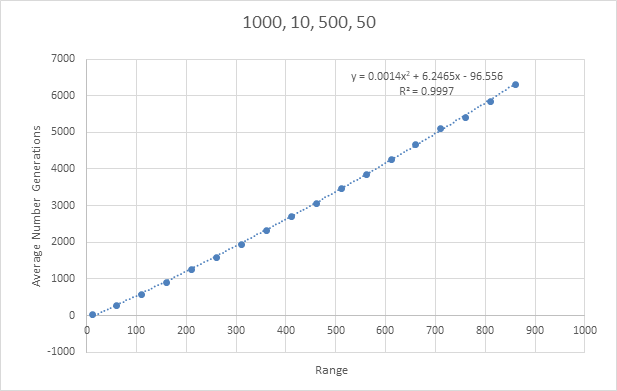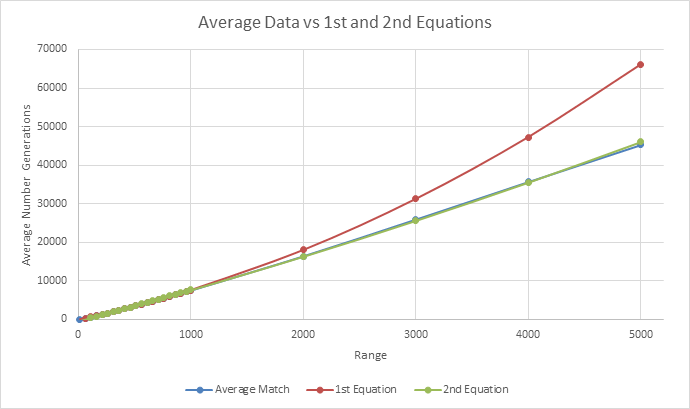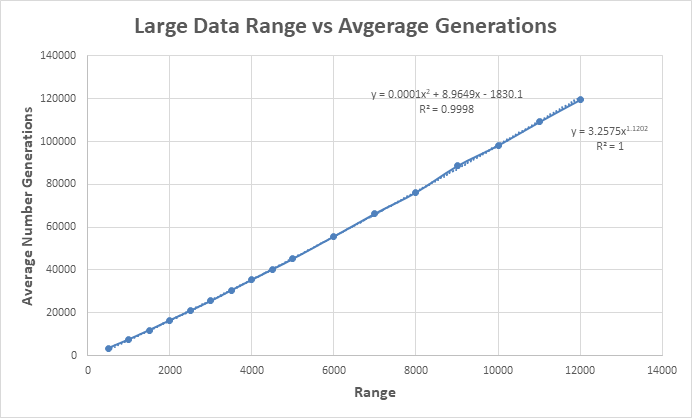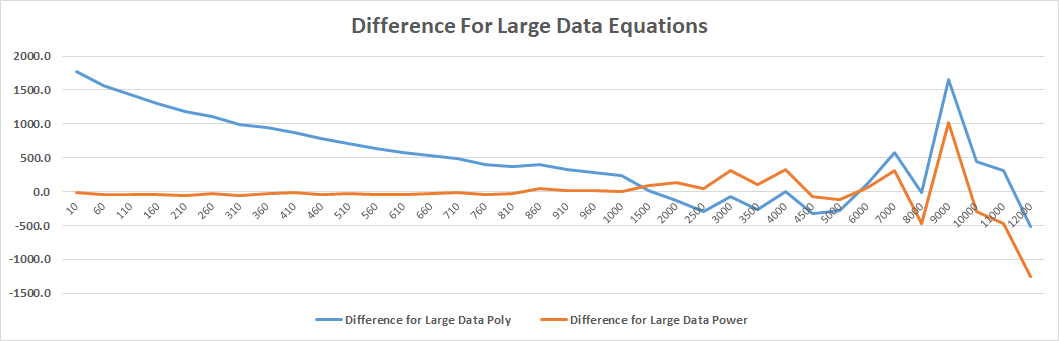# Randomly Generating Numbers to Fulfil an Integer Range

7 Dec 2017 Investigations pythonThis experiment looks at how many randoms numbers will need to be generated to fulfil an integer range. I use python to generate random numbers and simulate thousands of tests. This was conducted to find the range of 16,777,216 for an app I had developed.

## Introduction

I was recently discussing 'stupid' apps with a flatmate and how they do so well on app stores. We were thinking of really stupid apps and had come up with the idea of just changing the colour when a user tapped on the screen. Obviously, there would be more to the app but it seemed very achievable and something fun to make. My idea was to generate the colours as hexadecimal values and display them to the user along with the colour. I then thought about making the aim of the app to get all the colours; a user would randomly generate colours until they have gotten them all. With there being 16,777,216 different colours that can be represented using hexadecimal I noticed very quickly that this is very unachievable for a user, but how unachievable really was it?

## Plan

My plan to finding how 'unachievable' the problem is, is to generate random numbers in a range until all individual numbers in that range are generated. This will be done a few times for certain ranges to get averages and then will be plotted to get a line of best fit. With this line of best fit, I should be able to predict ranges much larger than I will be testing with.

## Creating An Initial Method

Sitting in my girlfriend's English lecture at Victoria University seemed like the best place to start this. The plan was to make a definition that took a range integer and randomly generates values until all values in the range were found. It would then return how many generations it took to get all values. To check when I had all values, I sorted the list using pythons built-in list sort and then checked if it was identical to a generated list containing 1 to the range in order which was created using the range function in list comprehension.

``````import random

def get_generation(r):
aim = [i for i in range(1, r + 1)]
generation_count = 0
generated = []
while True:
gen = random.randint(1, r)
generation_count += 1
if gen not in generated:
generated.append(gen)
generated.sort()
if generated == aim:
break
return generation_count

runs = 1000 # How many runs to get average
min_ = 10 # Start
max_ = 500 # End
step_ = 50 # Step

ranges_to_run = [i for i in range(min_, max_, step_)]
total = 0
for range_aim in ranges_to_run:
for i in range(runs):
total += get_generation(range_aim)

print (range_aim, "\t", total/runs)
total = 0
``````

This script was clean and was easy to use; all I had to do was change the four runs, min_, max_ and step_ variables to change looping for average calculation and the integers tested.

## Testing Small Numbers

Initially, this script was tested with 1000 runs, min_ of 10, max_ of 500 and a step_ of 50; the results are as follows.

Range Average Number Generations
10 29.522
60 280.669
110 580.739
160 910.13
210 1264.651
260 1600.558
310 1960.173
360 2326.071
410 2710.593
460 3069.874
510 3477.14
560 3854.053
610 4283.64
660 4683.23
710 5121.04
760 5414.062
810 5865.353
860 6321.91This data produced gives a clear pattern and produced a line of best fit with R^2 value of 0.9997 which is excellent. Using this equation to calculate the target value of 16,777,216 I got a value of 3.94x10^11. Even though this is a very large number, it doesn't seem like it is incorrect. There is a slight exponential addition to the equation calculated which will mean that little increases in x will give larger increases in y. With this looking like a great start I went and tested a couple of bigger numbers.

### Bigger Numbers

Due to the time to compute these values, I tested with larger numbers that weren't too much bigger than the previously tested numbers. I tested 1000, 2000, 3000, 4000 and 5000 and compared them to the expected value based on the last equation generated.

Range Average Number Generations Expected
1000 7469.096 7549.944
2000 16373.401 17996.44
3000 25888.327 31242.94
4000 35629.988 47289.44
5000 45216.905 66135.944

Looking at this data, the larger the numbers that were tested, the larger the difference between the actual and expected based on the last equation. After adding this data to the last data gathered and graphing it, the new equation is y = 0.0003x2 + 7.8024x - 470.14 with an R^2 of 0.9997. Even though this R^2 value is good, the lower values tested are far off the expected. An example of this difference for 10 being -33.951 for the last equation and -392.09 for this new equation even though the actual value sits around 29.735. This difference however, starts to decrease when the range is over 200.Even with this decrease being fixed, I am going to have to generate much larger ranges to have a chance of being able to predict the target value. Unfortunately, this isn't as easy as it seems as the current method is quite slow.

## Can Efficiency Be Improved?

My first thought to fix this time issue was "Is my method inefficient?". I ran the method for 1000 runs at a 2000 range using the PyCharm profiler as it ran. The results from the profiler are as follows.

Name Call Count Time (ms) Own Time (ms) Own Time %
get_generation 1000 282050 222018 78.71
<method 'sort' of 'list' objects> 2000000 29729 29729 10.54
randrange 16247313 24371 12351 4.38
_randbelow 16247313 12019 9285 3.29
randint 16247313 30102 5730 2.03
<method 'getrandbits' of '_random.Random' objects> 16636543 1972 1972 0.70
<method 'bit_length' of 'int' objects> 16247313 762 762 0.27
<method 'append' of 'list' objects> 2000000 134 134 0.05
<listcomp> 1000 66 66 0.02
<module> 1 282072 21 0.01
<listcomp> 1 0 0 0.00
<built-in method builtins.print> 1 0 0 0.00

From this we can see that only 21ms was spent outside the get_generation method which is good, nothing is causing efficiency issues in the function called and small calculations. Ignoring the get_generation method as a whole and looking at the smaller bits of it, we can see that the .sort() method used quite a bit of the time. 10.5% of the total execution time was just sorting the list. There is no issue with excessive calling as 2000*1000 is 2,000,000 which is what it's call count was. This shows that it might be ideal to substitute this with an alternative way of saying that the list is full and breaking.

One idea of substitution I had was to sort the list once it had the desired length and then check against the aim variable. After thinking a bit more I realised I didn't even need the sort function. Since I was making sure the random number generated wasn't in the list before inserting it, I can just check its length. If the length is the same as the amount of numbers it is trying to generate, then it can break. This will completely remove the .sort() method and hopefully reduce execution time and speed things up.

### Removing .sort()

I changed the get_generation method to get rid of the .sort() list function. This new method removes the need for a target list as it compares the length of the list to the target range. If both are equal this means that we have generated all the values in the range and can break out of the loop. This can be done as the list is checked before a value is added to make sure the value isn't in there.

``````def get_generation(r):
generation_count = 0
generated = []
while True:
gen = random.randint(1, r)
generation_count += 1
if gen not in generated:
generated.append(gen)
if len(generated) == r:
break
return generation_count
``````

The new results from the profiler while using this method for 1000 runs at a 2000 range are as follows.

Name Call Count Time (ms) Own Time (ms) Own Time %
get_generation 1000 308617 276438 89.56
randrange 16389852 25514 13030 4.22
_randbelow 16389852 12483 9620 3.12
randint 16389852 31797 6283 2.04
<method 'getrandbits' of '_random.Random' objects> 16783354 2073 2073 0.67
<method 'bit_length' of 'int' objects> 16389852 789 789 0.26
<built-in method builtins.len> 2000000 162 162 .05
<method 'append' of 'list' objects> 2000000 148 148 0.05
<listcomp> 1000 69 69 0.02
<module> 1 308647 29 0.01
<built-in method builtins.print> 1 0 0 0.00

Even though this method did take longer to run as shown in the <module> time, we have removed bulk of the .sort() method. This execution time increase would have been due to the fact different numbers were generated.

### Method to Test

To test the two methods, it would be best to 'pull them apart' and use the exact same numbers on them to see what sort of speed increase there really is. My plan is to generate random numbers in a certain range, make sure all numbers in the range are located in the list and then run them through the two methods. This will mean exactly the same data is being passed through to ensure a fairer test. I created a small script to generate random numbers in a range and stop when all the required numbers are present. I moved this method and created modified versions of the methods I was testing allowing them to take in the new list of numbers only. I then timed the two methods. The script and data are as follows.

``````import random
import time

def generate_testing_data(r):
generated = []
aim = [i for i in range(1, r+1)]
while True:
generated.append(random.randint(1, r))
if all([i in generated for i in aim]):
break
return generated

def test_new(r, numbers):
index = 0
generated = []
while True:
gen = numbers[index]
index += 1
if gen not in generated:
generated.append(gen)
if len(generated) == r:
break
return True

def test_old(r, numbers):
index = 0
aim = [i for i in range(1, r + 1)]
generated = []
while True:
gen = numbers[index]
index += 1
if gen not in generated:
generated.append(gen)
generated.sort()
if generated == aim:
break
return True

for i in range(10):
r = 1000
data = generate_testing_data(r)
start_time = time.time()
test_old(r, data)
old_time = time.time() - start_time
start_time = time.time()
test_new(r, data)
new_time = time.time() - start_time
print (r, "\t", len(data), "\t", old_time, "\t", new_time)
``````

### Results

I ran this 16 times and recorded the results. All the ranges are 1000 but due to the generation of random numbers, the lengths will be different.

Length Old Method (ms) New Method(ms) Difference Gained
10884 0.083470821 0.089237928 -0.005767107
8740 0.06921196 0.075702429 -0.006490469
8144 0.061194181 0.06467104 -0.003476858
8067 0.059659004 0.062694788 -0.003035784
8031 0.059160709 0.062134027 -0.002973318
7937 0.059129238 0.061220646 -0.002091408
7579 0.056650877 0.060160398 -0.003509521
7572 0.055316925 0.060691118 -0.005374193
7458 0.061327457 0.063675165 -0.002347708
7411 0.060157061 0.061663151 -0.00150609
7273 0.054145336 0.055227518 -0.001082182
7124 0.055146694 0.057681561 -0.002534866
7041 0.052210808 0.053673506 -0.001462698
7018 0.052706957 0.056149721 -0.003442764
5575 0.042111397 0.043116093 -0.001004696
5539 0.045147181 0.044615984 0.000531197
10884 0.083470821 0.089237928 -0.005767107

Unfortunately, these results weren't as good as I had hoped. All but one of the differences are negative, meaning this new method increased the time it took to compute the calculations required.

Since this method didn't speed processing up (and if it did, not by much), I decided to turn to threading. Even with its notorious name in Python, I have done a few projects using python threading. To use threading, I plan to call the get_generation method allowing a certain amount of threads at once (can be changed). When a thread completes, I want to put the data in a list somewhere and when all threads for a range is complete, add the data to a CSV file. This can then be used to look at the data with more depth than previous.

### Method

After some quick planning, I completed the new script to how I had planned. Some quick testing and formatting of the CSV output then had it ready to be fully run.

This did in-fact speed up the process of getting the data but threads were not called as fast as expected and it still did have difficulty on higher numbers. To give a bit of feedback I added a method to look at what was running and provide more useful messages. With this output, I noted that threads were peaking at just over 270, this wasn't currently an issue, just interesting. This was the final script for the threading version.

``````import threading
import random

def __init__(self, range_, return_class, id):
self.range_ = range_
self.return_class = return_class
self.id = id

def run(self):
status("Starting " + self.id)
aim = [i for i in range(1, self.range_ + 1)]
generation_count = 0
generated = []
while True:
gen = random.randint(1, self.range_)
generation_count += 1
if gen not in generated:
generated.append(gen)
generated.sort()
if generated == aim:
break
self.return_class.put(self.range_, generation_count)
status("Stopping " + self.id)

def __init__(self, range_values, runs):
self.data = {str(i):[] for i in range_values}
self.runs = runs

def put(self, range_, generation_count):
self.data[str(range_)].append(str(generation_count))
if len(self.data[str(range_)]) >= self.runs:
self.save(range_)
status("Saved " + str(range_))

def save(self, range_):
f = open('output.csv', 'a')
f.write(str(range_) + ',' + ','.join(self.data[str(range_)]) + "\n")
f.close()

def status(message=""):
continue
else:
print (str(thread_data) + "\t" + message)

runs = 1000
range_values = [i for i in range(500, 10001, 500)]

for range_value in range_values:
status("Starting " + str(range_value))
for i in range(runs):
while True:
id = str(range_value) + "_" + str(i)
break
status("Started " + str(range_value))
``````

### Results

Once again, the bigger the range, the longer it took to compute. Even with threads, 6000 was a stretch. I decided to bring down the run number to 200 to test some bigger numbers; this will be reflected in the standard error. I understand this will give less accurate data but hopefully, it will be accurate enough to give a reasonable line of best fit.

I compared the threading with the original method but running them both at the same time. Interestingly it wasn't that much faster as the threading had completed when the original method had completed 196/200. I will still use the threading method as it has a better output and gives more information on the current status.

Now having the raw data, I was able to calculate the average, min, max and standard error of each of the samples. Using excel I simply just inserted formulas and then took in the row of data. The data is as follows.

Range Runs Average Min Max Sth. Err.
500 1000 3392.84 2049 7147 21.42
1000 1000 7471.59 5059 13448 38.72
1500 1000 11852.733 8109 25614 60.39
2000 1000 16449.733 10935 31156 84.25
2500 1000 20905.963 14183 34315 96.27
3000 1000 25500.513 17861 45517 113.56
3500 1000 30508.723 22411 61916 137.92
4000 1000 35431.821 25825 63598 161.75
4500 1000 40217.431 28229 64413 176.81
5000 1000 45216.391 33309 77804 200.87
6000 200 55676.045 42055 84056 548.92
7000 200 66404.76 49262 109629 661.75
8000 200 76281.205 58873 121843 740.33
9000 200 88607.415 68325 123956 800.37
10000 200 98259.72 76908 182110 1012.62
11000 200 109195.375 84138 172973 1047.34
12000 200 119634.365 88378 162256 989.33From this data, I discovered that a trendline formula with a power had developed a significant R^2 value and fits visually. I also once again obtained a polynomial function looking similar to the last. These new equations were y = 3.2575x^1.1202 and y = 0.0001x^2 + 8.9649x - 1830.1.

## Equation Analysis

I now have four equations being:

• 1st Equation: y = 0.0014x^2 + 6.2465x - 96.556
• 2nd Equation: y = 0.0003x^2 + 7.8024x - 470.14
• Large Data Power: y = 3.2575x^1.1202
• Large Data Polynomial: y = 0.0001x^2 + 8.9649x - 1830.1

With these, I can now take all the data that has been generated and compare it to each of these equations.

Range Runs Average Match 1st Equation 2nd Equation Large Data Power Large Data Polynomial
10 1000 29.7 -34.0 -392.1 -1740.4 43.0
60 1000 278.4 283.3 -0.9 -1291.8 319.7
110 1000 584.6 607.5 391.7 -842.8 630.5
160 1000 910.5 938.7 785.9 -393.2 959.3
210 1000 1240.0 1276.9 1181.5 56.9 1300.9
260 1000 1618.5 1622.2 1578.7 507.5 1652.5
310 1000 1953.6 1974.4 1977.3 958.6 2012.4
360 1000 2355.6 2333.6 2377.5 1410.2 2379.3
410 1000 2731.2 2699.8 2779.1 1862.3 2752.5
460 1000 3092.8 3073.1 3182.3 2314.9 3131.2
510 1000 3482.8 3453.3 3586.9 2768.0 3514.8
560 1000 3862.0 3840.5 3993.1 3221.6 3903.1
610 1000 4256.9 4234.7 4400.7 3675.7 4295.5
660 1000 4669.0 4636.0 4809.9 4130.3 4691.8
710 1000 5070.3 5044.2 5220.5 4585.4 5091.7
760 1000 5444.0 5459.4 5632.7 5041.0 5495.1
810 1000 5868.0 5881.6 6046.3 5497.1 5901.6
860 1000 6356.6 6310.9 6461.5 5953.7 6311.2
910 1000 6736.2 6747.1 6878.1 6410.8 6723.6
960 1000 7147.9 7190.3 7296.3 6868.4 7138.8
1000 1000 7469.1 7549.9 7631.9 7234.8 7472.8
1500 1000 11852.7 12423.2 11907.9 11842.3 11769.1
2000 1000 16373.4 17996.4 16333.9 16499.7 16244.2
2500 1000 20906.0 24269.7 20909.9 21207.2 20857.2
3000 1000 25888.3 31242.9 25635.9 25964.6 25583.2
3500 1000 30508.7 38916.2 30511.9 30772.1 30405.3
4000 1000 35630.0 47289.4 35537.9 35629.5 35311.2
4500 1000 40217.4 56362.7 40713.9 40537.0 40291.5
5000 1000 45216.9 66135.9 46039.9 45494.4 45338.9
6000 200 55676.0 87782.4 57141.9 55559.3 55612.1
7000 200 66404.8 112228.9 68843.9 65824.2 66094.2
8000 200 76281.2 139475.4 81145.9 76289.1 76758.4
9000 200 88607.4 169521.9 94047.9 86954.0 87584.4
10000 200 98259.7 202368.4 107549.9 97818.9 98556.3
11000 200 109195.4 238014.9 121651.9 108883.8 109661.1
12000 200 119634.4 276461.4 136353.9 120148.7 120888.0

Looking at this table, we can see that the two large data equations have a much better guess to the actual values than the previous two equations. This is because the equations have been formed with more data allowing them to be able to guess larger values more accurately. I will then compare the difference between the average values and the last two equations on a graph.## Conclusion

By looking at the graph, it appears that the large data polynomial equation was the best at predicting the averages. At about 1500 the equations start to be a bit unreliable but are still staying reasonable close being at most 13% in extreme cases away from the average. This would be because I didn't do enough tests to calculate averages and this theory definitely shows when the tests go above a range of 6000 as that is where I only calculated averages based off 200 runs due to the time to run the tests.

Thus, from these tests, I believe the most reliable equation to test the range of 16,777,216 with is y = 0.0001x^2 + 8.9649x - 1830.1.

And then the calculation; y = 0.0001(16777216)^2 + 8.9649(16777216) - 1830.1, y = 28,297,901,904.684002

28 and a quarter billion is still a very big number but a large number was expected. If someone could keep a rate of 400 taps per minute (it would get much slower over time but this is theoretical), it would take them over 134 and a half years to reach this number on average. Even if someone got lucky and had little duplicates compared to an average, I feel this game (Colour) would still not be possible to achieve in anyone's lifetime currently.